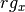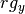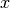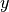Shortcuts

# Spearman Corr. Coef.¶

## Module Interface¶

class torchmetrics.SpearmanCorrCoef(num_outputs=1, **kwargs)[source]

whereandare the rank associated to the variablesand. Spearmans correlations coefficient corresponds to the standard pearsons correlation coefficient calculated on the rank variables.

As input to forward and update the metric accepts the following input:

• preds (Tensor): Predictions from model in float tensor with shape (N,d)

• target (Tensor): Ground truth values in float tensor with shape (N,d)

As output of forward and compute the metric returns the following output:

Parameters
Example (single output regression):
>>> from torchmetrics import SpearmanCorrCoef
>>> target = torch.tensor([3, -0.5, 2, 7])
>>> preds = torch.tensor([2.5, 0.0, 2, 8])
>>> spearman = SpearmanCorrCoef()
>>> spearman(preds, target)
tensor(1.0000)

Example (multi output regression):
>>> from torchmetrics import SpearmanCorrCoef
>>> target = torch.tensor([[3, -0.5], [2, 7]])
>>> preds = torch.tensor([[2.5, 0.0], [2, 8]])
>>> spearman = SpearmanCorrCoef(num_outputs=2)
>>> spearman(preds, target)
tensor([1.0000, 1.0000])


Initializes internal Module state, shared by both nn.Module and ScriptModule.

## Functional Interface¶

torchmetrics.functional.spearman_corrcoef(preds, target)[source]

whereandare the rank associated to the variables x and y. Spearmans correlations coefficient corresponds to the standard pearsons correlation coefficient calculated on the rank variables.

Parameters
Example (single output regression):
>>> from torchmetrics.functional import spearman_corrcoef
>>> target = torch.tensor([3, -0.5, 2, 7])
>>> preds = torch.tensor([2.5, 0.0, 2, 8])
>>> spearman_corrcoef(preds, target)
tensor(1.0000)

Example (multi output regression):
>>> from torchmetrics.functional import spearman_corrcoef
>>> target = torch.tensor([[3, -0.5], [2, 7]])
>>> preds = torch.tensor([[2.5, 0.0], [2, 8]])
>>> spearman_corrcoef(preds, target)
tensor([1.0000, 1.0000])

Return type

Tensor

© Copyright Copyright (c) 2020-2023, Lightning-AI et al... Revision 6853c553.

Built with Sphinx using a theme provided by Read the Docs.
Versions
latest
stable
v0.11.2
v0.11.1
v0.11.0
v0.10.3
v0.10.2
v0.10.1
v0.10.0
v0.9.3
v0.9.2
v0.9.1
v0.9.0
v0.8.2
v0.8.1
v0.8.0
v0.7.3
v0.7.2
v0.7.1
v0.7.0
v0.6.2
v0.6.1
v0.6.0
v0.5.1
v0.5.0
v0.4.1
v0.4.0
v0.3.2
v0.3.1
v0.3.0
v0.2.0
v0.1.0